Open in App
Not now

## Related Articles

• Write an Interview Experience

• Difficulty Level : Easy
• Last Updated : 25 Jan, 2023

A linked list is a linear data structure, in which the elements are not stored at contiguous memory locations. The elements in a linked list are linked using pointers. In simple words, a linked list consists of nodes where each node contains a data field and a reference(link) to the next node in the list.

It is the simplest type of linked list in which every node contains some data and a pointer to the next node of the same data type.

The node contains a pointer to the next node means that the node stores the address of the next node in the sequence. A single linked list allows the traversal of data only in one way. Below is the image for the same: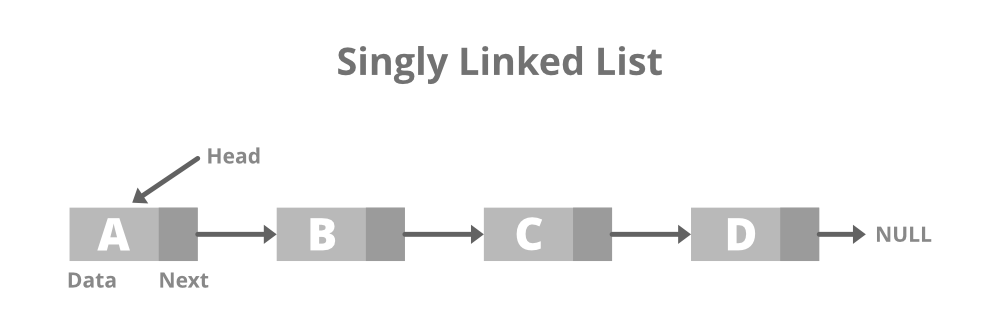Below is the structure of the singly linked list

## C++

 `// Node of a singly linked list``class` `Node {``public``:``    ``int` `data;` `    ``// Pointer to next node in LL``    ``Node* next;``};`

## Java

 `// Node of a singly linked list``static` `class` `Node {``    ``int` `data;` `    ``// Pointer to next node in LL``    ``Node next;``};` `// this code is contributed by shivani`

## Python3

 `# Node of a singly linked list``class` `Node:``    ``def` `__init__(``self``, data):``        ``self``.data ``=` `data``        ``self``.``next` `=` `None`

## C#

 `// Structure of a singly linked list Node``public` `class` `Node {``    ``public` `int` `data;` `    ``// Pointer to next node in LL``    ``public` `Node next;``};` `// this code is contributed by shivanisinghss2110`

## Javascript

 `// Node of a singly linked list``class Node``{``    ``constructor()``    ``{``        ``this``.data=0;``        ` `        ``// Pointer to next node``        ``this``.next=``null``;``    ``}``}` `// This code is contributed by SHUBHAMSINGH10`

## C++

 `// C++ program to illustrate creation``// and traversal of Singly Linked List` `#include ``using` `namespace` `std;` `// Structure of Node``class` `Node {``public``:``    ``int` `data;``    ``Node* next;``};` `// Function to print the content of``// linked list starting from the``// given node``void` `printList(Node* n)``{` `    ``// Iterate till n reaches NULL``    ``while` `(n != NULL) {` `        ``// Print the data``        ``cout << n->data << ``" "``;``        ``n = n->next;``    ``}``}` `// Driver Code``int` `main()``{``    ``Node* head = NULL;``    ``Node* second = NULL;``    ``Node* third = NULL;` `    ``// Allocate 3 nodes in the heap``    ``head = ``new` `Node();``    ``second = ``new` `Node();``    ``third = ``new` `Node();` `    ``// Assign data in first node``    ``head->data = 1;` `    ``// Link first node with second``    ``head->next = second;` `    ``// Assign data to second node``    ``second->data = 2;``    ``second->next = third;` `    ``// Assign data to third node``    ``third->data = 3;``    ``third->next = NULL;` `    ``printList(head);` `    ``return` `0;``}`

## Java

 `// Java program to illustrate``// creation and traversal of``// Singly Linked List``class` `GFG {` `    ``// Structure of Node``    ``static` `class` `Node {``        ``int` `data;``        ``Node next;``    ``};` `    ``// Function to print the content of``    ``// linked list starting from the``    ``// given node``    ``static` `void` `printList(Node n)``    ``{``        ``// Iterate till n reaches null``        ``while` `(n != ``null``) {``            ``// Print the data``            ``System.out.print(n.data + ``" "``);``            ``n = n.next;``        ``}``    ``}` `    ``// Driver Code``    ``public` `static` `void` `main(String[] args)``    ``{``        ``Node head = ``null``;``        ``Node second = ``null``;``        ``Node third = ``null``;` `        ``// Allocate 3 nodes in``        ``// the heap``        ``head = ``new` `Node();``        ``second = ``new` `Node();``        ``third = ``new` `Node();` `        ``// Assign data in first``        ``// node``        ``head.data = ``1``;` `        ``// Link first node with``        ``// second``        ``head.next = second;` `        ``// Assign data to second``        ``// node``        ``second.data = ``2``;``        ``second.next = third;` `        ``// Assign data to third``        ``// node``        ``third.data = ``3``;``        ``third.next = ``null``;` `        ``printList(head);``    ``}``}` `// This code is contributed by Princi Singh`

## C#

 `// C# program to illustrate``// creation and traversal of``// Singly Linked List``using` `System;` `class` `GFG {` `    ``// Structure of Node``    ``public` `class` `Node {``        ``public` `int` `data;``        ``public` `Node next;``    ``};` `    ``// Function to print the content of``    ``// linked list starting from the``    ``// given node``    ``static` `void` `printList(Node n)``    ``{` `        ``// Iterate till n reaches null``        ``while` `(n != ``null``) {` `            ``// Print the data``            ``Console.Write(n.data + ``" "``);``            ``n = n.next;``        ``}``    ``}` `    ``// Driver Code``    ``public` `static` `void` `Main(String[] args)``    ``{``        ``Node head = ``null``;``        ``Node second = ``null``;``        ``Node third = ``null``;` `        ``// Allocate 3 nodes in``        ``// the heap``        ``head = ``new` `Node();``        ``second = ``new` `Node();``        ``third = ``new` `Node();` `        ``// Assign data in first``        ``// node``        ``head.data = 1;` `        ``// Link first node with``        ``// second``        ``head.next = second;` `        ``// Assign data to second``        ``// node``        ``second.data = 2;``        ``second.next = third;` `        ``// Assign data to third``        ``// node``        ``third.data = 3;``        ``third.next = ``null``;` `        ``printList(head);``    ``}``}` `// This code is contributed by Amit Katiyar`

## Python3

 `# structure of Node``class` `Node:``    ``def` `__init__(``self``, data):``        ``self``.data ``=` `data``        ``self``.``next` `=` `None`  `class` `LinkedList:``    ``def` `__init__(``self``):``        ``self``.head ``=` `None``        ``self``.last_node ``=` `None` `    ``# function to add elements to linked list``    ``def` `append(``self``, data):``        ``# if linked list is empty then last_node will be none so in if condition head will be created``        ``if` `self``.last_node ``is` `None``:``            ``self``.head ``=` `Node(data)``            ``self``.last_node ``=` `self``.head``        ``# adding node to the tail of linked list``        ``else``:``            ``self``.last_node.``next` `=` `Node(data)``            ``self``.last_node ``=` `self``.last_node.``next` `# function to print the content of linked list``    ``def` `display(``self``):``        ``current ``=` `self``.head``      ``# traversing the linked list``        ``while` `current ``is` `not` `None``:``          ``# at each node printing its data``            ``print``(current.data, end``=``' '``)``           ``# giving current next node``            ``current ``=` `current.``next``        ``print``()` `# Driver code``if` `__name__ ``=``=` `'__main__'``:``    ``L ``=` `LinkedList()``    ``# adding elements to the linked list``    ``L.append(``1``)``    ``L.append(``2``)``    ``L.append(``3``)``    ``# displaying elements of linked list``    ``L.display()`

## Javascript

 `// JavaScript program to illustrate``// creation and traversal of``// Singly Linked List` `// Structure of Node``class Node``{``    ``constructor()``    ``{``        ``this``.data=0;``        ``this``.next=``null``;``    ``}``}` `// Function to print the content of``// linked list starting from the``// given node``function` `printList(n)``{``    ``// Iterate till n reaches null``  ``while` `(n != ``null``)``  ``{``    ``// Print the data``    ``document.write(n.data + ``" "``);``    ``n = n.next;``  ``}``}` `// Driver Code``let head = ``null``;``let second = ``null``;``let third = ``null``;` `// Allocate 3 nodes in``// the heap``head = ``new` `Node();``second = ``new` `Node();``third = ``new` `Node();` `// Assign data in first``// node``head.data = 1;` `// Link first node with``// second``head.next = second;` `// Assign data to second``// node``second.data = 2;``second.next = third;` `// Assign data to third``// node``third.data = 3;``third.next = ``null``;` `printList(head);`  `// This code is contributed by unknown2108`

Output

`1 2 3 `

Time Complexity: O(N)
Auxiliary Space: O(N)

A doubly linked list or a two-way linked list is a more complex type of linked list that contains a pointer to the next as well as the previous node in sequence.

Therefore, it contains three parts of data, a pointer to the next node, and a pointer to the previous node. This would enable us to traverse the list in the backward direction as well. Below is the image for the same: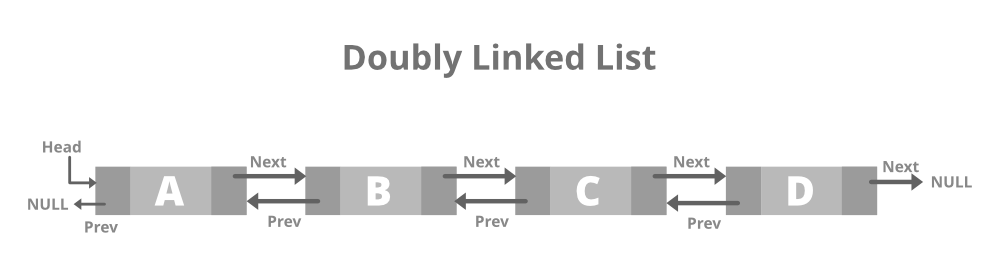## C++

 `// Node of a doubly linked list``struct` `Node {``    ``int` `data;` `    ``// Pointer to next node in DLL``    ``struct` `Node* next;` `    ``// Pointer to the previous node in DLL``    ``struct` `Node* prev;``};`

## Java

 `// Doubly linked list``// node``static` `class` `Node {``    ``int` `data;` `    ``// Pointer to next node in DLL``    ``Node next;` `    ``// Pointer to the previous node in DLL``    ``Node prev;``};` `// This code is contributed by shivani`

## Python3

 `# structure of Node``class` `Node:``    ``def` `__init__(``self``, data):``        ``self``.previous ``=` `None``        ``self``.data ``=` `data``        ``self``.``next` `=` `None`

## C#

 `// Doubly linked list``// node``public` `class` `Node {``    ``public` `int` `data;` `    ``// Pointer to next node in DLL``    ``public` `Node next;` `    ``// Pointer to the previous node in DLL``    ``public` `Node prev;``};` `// This code is contributed by shivanisinghss2110`

## Javascript

 ``

## C++

 `// C++ program to illustrate creation``// and traversal of Doubly Linked List` `#include ``using` `namespace` `std;` `// Doubly linked list node``class` `Node {``public``:``    ``int` `data;``    ``Node* next;``    ``Node* prev;``};` `// Function to push a new element in``// the Doubly Linked List``void` `push(Node** head_ref, ``int` `new_data)``{``    ``// Allocate node``    ``Node* new_node = ``new` `Node();` `    ``// Put in the data``    ``new_node->data = new_data;` `    ``// Make next of new node as``    ``// head and previous as NULL``    ``new_node->next = (*head_ref);``    ``new_node->prev = NULL;` `    ``// Change prev of head node to``    ``// the new node``    ``if` `((*head_ref) != NULL)``        ``(*head_ref)->prev = new_node;` `    ``// Move the head to point to``    ``// the new node``    ``(*head_ref) = new_node;``}` `// Function to traverse the Doubly LL``// in the forward & backward direction``void` `printList(Node* node)``{``    ``Node* last;` `    ``cout << ``"\nTraversal in forward"``         ``<< ``" direction \n"``;``    ``while` `(node != NULL) {` `        ``// Print the data``        ``cout << ``" "` `<< node->data << ``" "``;``        ``last = node;``        ``node = node->next;``    ``}` `    ``cout << ``"\nTraversal in reverse"``         ``<< ``" direction \n"``;``    ``while` `(last != NULL) {` `        ``// Print the data``        ``cout << ``" "` `<< last->data << ``" "``;``        ``last = last->prev;``    ``}``}` `// Driver Code``int` `main()``{``    ``// Start with the empty list``    ``Node* head = NULL;` `    ``// Insert 6.``    ``// So linked list becomes 6->NULL``    ``push(&head, 6);` `    ``// Insert 7 at the beginning. So``    ``// linked list becomes 7->6->NULL``    ``push(&head, 7);` `    ``// Insert 1 at the beginning. So``    ``// linked list becomes 1->7->6->NULL``    ``push(&head, 1);` `    ``cout << ``"Created DLL is: "``;``    ``printList(head);` `    ``return` `0;``}`

## Java

 `// Java program to illustrate``// creation and traversal of``// Doubly Linked List` `import` `java.util.*;``class` `GFG {` `    ``// Doubly linked list``    ``// node``    ``static` `class` `Node {``        ``int` `data;``        ``Node next;``        ``Node prev;``    ``};` `    ``static` `Node head_ref;` `    ``// Function to push a new``    ``// element in the Doubly``    ``// Linked List``    ``static` `void` `push(``int` `new_data)``    ``{``        ``// Allocate node``        ``Node new_node = ``new` `Node();` `        ``// Put in the data``        ``new_node.data = new_data;` `        ``// Make next of new node as``        ``// head and previous as null``        ``new_node.next = head_ref;``        ``new_node.prev = ``null``;` `        ``// Change prev of head node to``        ``// the new node``        ``if` `(head_ref != ``null``)``            ``head_ref.prev = new_node;` `        ``// Move the head to point to``        ``// the new node``        ``head_ref = new_node;``    ``}` `    ``// Function to traverse the``    ``// Doubly LL in the forward``    ``// & backward direction``    ``static` `void` `printList(Node node)``    ``{``        ``Node last = ``null``;` `        ``System.out.print(``"\nTraversal in forward"``                         ``+ ``" direction \n"``);``        ``while` `(node != ``null``) {``            ``// Print the data``            ``System.out.print(``" "` `+ node.data + ``" "``);``            ``last = node;``            ``node = node.next;``        ``}` `        ``System.out.print(``"\nTraversal in reverse"``                         ``+ ``" direction \n"``);` `        ``while` `(last != ``null``) {``            ``// Print the data``            ``System.out.print(``" "` `+ last.data + ``" "``);``            ``last = last.prev;``        ``}``    ``}` `    ``// Driver Code``    ``public` `static` `void` `main(String[] args)``    ``{``        ``// Start with the empty list``        ``head_ref = ``null``;` `        ``// Insert 6.``        ``// So linked list becomes``        ``// 6.null``        ``push(``6``);` `        ``// Insert 7 at the beginning.``        ``// So linked list becomes``        ``// 7.6.null``        ``push(``7``);` `        ``// Insert 1 at the beginning.``        ``// So linked list becomes``        ``// 1.7.6.null``        ``push(``1``);` `        ``System.out.print(``"Created DLL is: "``);``        ``printList(head_ref);``    ``}``}` `// This code is contributed by Princi Singh`

## Python3

 `# Python3 program to illustrate``# creation and traversal of``# Doubly Linked List` `# structure of Node``class` `Node:``    ``def` `__init__(``self``, data):``        ``self``.previous ``=` `None``        ``self``.data ``=` `data``        ``self``.``next` `=` `None`  `class` `DoublyLinkedList:``    ``def` `__init__(``self``):``        ``self``.head ``=` `None``        ``self``.start_node ``=` `None``        ``self``.last_node ``=` `None` `    ``# function to add elements to doubly linked list``    ``def` `append(``self``, data):``        ``# is doubly linked list is empty then last_node will be none so in if condition head will be created``        ``if` `self``.last_node ``is` `None``:``            ``self``.head ``=` `Node(data)``            ``self``.last_node ``=` `self``.head``        ``# adding node to the tail of doubly linked list``        ``else``:``            ``new_node ``=` `Node(data)``            ``self``.last_node.``next` `=` `new_node``            ``new_node.previous ``=` `self``.last_node``            ``new_node.``next` `=` `None``            ``self``.last_node ``=` `new_node` `    ``# function to printing and traversing the content of doubly linked list from left to right and right to left``    ``def` `display(``self``, ``Type``):``        ``if` `Type` `=``=` `'Left_To_Right'``:``            ``current ``=` `self``.head``            ``while` `current ``is` `not` `None``:``                ``print``(current.data, end``=``' '``)``                ``current ``=` `current.``next``            ``print``()``        ``else``:``            ``current ``=` `self``.last_node``            ``while` `current ``is` `not` `None``:``                ``print``(current.data, end``=``' '``)``                ``current ``=` `current.previous``            ``print``()` `# Driver code``if` `__name__ ``=``=` `'__main__'``:``    ``L ``=` `DoublyLinkedList()``    ``L.append(``1``)``    ``L.append(``2``)``    ``L.append(``3``)``    ``L.append(``4``)``    ``L.display(``'Left_To_Right'``)``    ``L.display(``'Right_To_Left'``)`

## C#

 `// C# program to illustrate``// creation and traversal of``// Doubly Linked List` `using` `System;` `class` `GFG {` `    ``// Doubly linked list``    ``// node``    ``public` `class` `Node {``        ``public` `int` `data;``        ``public` `Node next;``        ``public` `Node prev;``    ``};` `    ``static` `Node head_ref;` `    ``// Function to push a new``    ``// element in the Doubly``    ``// Linked List``    ``static` `void` `push(``int` `new_data)``    ``{` `        ``// Allocate node``        ``Node new_node = ``new` `Node();` `        ``// Put in the data``        ``new_node.data = new_data;` `        ``// Make next of new node as``        ``// head and previous as null``        ``new_node.next = head_ref;``        ``new_node.prev = ``null``;` `        ``// Change prev of head node to``        ``// the new node``        ``if` `(head_ref != ``null``)``            ``head_ref.prev = new_node;` `        ``// Move the head to point to``        ``// the new node``        ``head_ref = new_node;``    ``}` `    ``// Function to traverse the``    ``// Doubly LL in the forward``    ``// & backward direction``    ``static` `void` `printList(Node node)``    ``{``        ``Node last = ``null``;` `        ``Console.Write(``"\nTraversal in forward"``                      ``+ ``" direction \n"``);` `        ``while` `(node != ``null``) {` `            ``// Print the data``            ``Console.Write(``" "` `+ node.data + ``" "``);``            ``last = node;``            ``node = node.next;``        ``}` `        ``Console.Write(``"\nTraversal in reverse"``                      ``+ ``" direction \n"``);` `        ``while` `(last != ``null``) {` `            ``// Print the data``            ``Console.Write(``" "` `+ last.data + ``" "``);``            ``last = last.prev;``        ``}``    ``}` `    ``// Driver Code``    ``public` `static` `void` `Main(String[] args)``    ``{` `        ``// Start with the empty list``        ``head_ref = ``null``;` `        ``// Insert 6.``        ``// So linked list becomes``        ``// 6.null``        ``push(6);` `        ``// Insert 7 at the beginning.``        ``// So linked list becomes``        ``// 7.6.null``        ``push(7);` `        ``// Insert 1 at the beginning.``        ``// So linked list becomes``        ``// 1.7.6.null``        ``push(1);` `        ``Console.Write(``"Created DLL is: "``);``        ``printList(head_ref);``    ``}``}` `// This code is contributed by Amit Katiyar`

## Javascript

 ``

Output

```Created DLL is:
Traversal in forward direction
1  7  6
Traversal in reverse direction
6  7  1```

A circular linked list is that in which the last node contains the pointer to the first node of the list.

While traversing a circular linked list, we can begin at any node and traverse the list in any direction forward and backward until we reach the same node we started. Thus, a circular linked list has no beginning and no end. Below is the image for the same: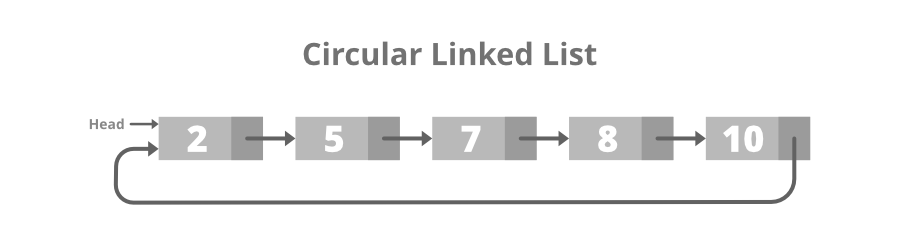Below is the structure of the Circular Linked List:

## C++

 `// Structure for a node``class` `Node {``public``:``    ``int` `data;` `    ``// Pointer to next node in CLL``    ``Node* next;``};`

## Java

 `// Structure for a node``static` `class` `Node {``    ``int` `data;` `    ``// Pointer to next node in CLL``    ``Node next;``};` `// This code is contributed by shivanisinghss2110`

## Python3

 `# structure of Node``class` `Node:``    ``def` `__init__(``self``, data):``        ``self``.data ``=` `data``        ``self``.``next` `=` `None`

## C#

 `// Structure for a node``public` `class` `Node {``    ``public` `int` `data;` `    ``// Pointer to next node in CLL``    ``public` `Node next;``};` `// This code is contributed by shivanisinghss2110`

## Javascript

 ``

## C++

 `// C++ program to illustrate creation``// and traversal of Circular LL` `#include ``using` `namespace` `std;` `// Structure for a node``class` `Node {``public``:``    ``int` `data;``    ``Node* next;``};` `// Function to insert a node at the``// beginning of Circular LL``void` `push(Node** head_ref, ``int` `data)``{``    ``Node* ptr1 = ``new` `Node();``    ``Node* temp = *head_ref;``    ``ptr1->data = data;``    ``ptr1->next = *head_ref;` `    ``// If linked list is not NULL then``    ``// set the next of last node``    ``if` `(*head_ref != NULL) {``        ``while` `(temp->next != *head_ref) {``            ``temp = temp->next;``        ``}``        ``temp->next = ptr1;``    ``}` `    ``// For the first node``    ``else``        ``ptr1->next = ptr1;` `    ``*head_ref = ptr1;``}` `// Function to print nodes in the``// Circular Linked List``void` `printList(Node* head)``{``    ``Node* temp = head;``    ``if` `(head != NULL) {``        ``do` `{` `            ``// Print the data``            ``cout << temp->data << ``" "``;``            ``temp = temp->next;``        ``} ``while` `(temp != head);``    ``}``}` `// Driver Code``int` `main()``{``    ``// Initialize list as empty``    ``Node* head = NULL;` `    ``// Created linked list will``    ``// be 11->2->56->12``    ``push(&head, 12);``    ``push(&head, 56);``    ``push(&head, 2);``    ``push(&head, 11);` `    ``cout << ``"Contents of Circular"``         ``<< ``" Linked List\n "``;``  ` `      ``// Function call``    ``printList(head);` `    ``return` `0;``}`

## Java

 `// Java program to illustrate``// creation and traversal of``// Circular LL` `import` `java.util.*;``class` `GFG {` `    ``// Structure for a``    ``// node``    ``static` `class` `Node {``        ``int` `data;``        ``Node next;``    ``};` `    ``// Function to insert a node``    ``// at the beginning of Circular``    ``// LL``    ``static` `Node push(Node head_ref, ``int` `data)``    ``{``        ``Node ptr1 = ``new` `Node();``        ``Node temp = head_ref;``        ``ptr1.data = data;``        ``ptr1.next = head_ref;` `        ``// If linked list is not``        ``// null then set the next``        ``// of last node``        ``if` `(head_ref != ``null``) {``            ``while` `(temp.next != head_ref) {``                ``temp = temp.next;``            ``}``            ``temp.next = ptr1;``        ``}` `        ``// For the first node``        ``else``            ``ptr1.next = ptr1;` `        ``head_ref = ptr1;``        ``return` `head_ref;``    ``}` `    ``// Function to print nodes in``    ``// the Circular Linked List``    ``static` `void` `printList(Node head)``    ``{``        ``Node temp = head;``        ``if` `(head != ``null``) {``            ``do` `{``                ``// Print the data``                ``System.out.print(temp.data + ``" "``);``                ``temp = temp.next;``            ``} ``while` `(temp != head);``        ``}``    ``}` `    ``// Driver Code``    ``public` `static` `void` `main(String[] args)``    ``{``        ``// Initialize list as empty``        ``Node head = ``null``;` `        ``// Created linked list will``        ``// be 11.2.56.12``        ``head = push(head, ``12``);``        ``head = push(head, ``56``);``        ``head = push(head, ``2``);``        ``head = push(head, ``11``);` `        ``System.out.print(``"Contents of Circular"``                         ``+ ``" Linked List\n "``);``      ` `        ``// Function call``        ``printList(head);``    ``}``}` `// This code is contributed by gauravrajput1`

## Python3

 `# Python3 program to illustrate``# creation and traversal of``# Circular LL` `# structure of Node``class` `Node:``    ``def` `__init__(``self``, data):``        ``self``.data ``=` `data``        ``self``.``next` `=` `None`  `class` `CircularLinkedList:``    ``def` `__init__(``self``):``        ``self``.head ``=` `None``        ``self``.last_node ``=` `None` `    ``# function to add elements to circular linked list``    ``def` `append(``self``, data):``        ``# is circular linked list is empty then last_node will be none so in if condition head will be created``        ``if` `self``.last_node ``is` `None``:``            ``self``.head ``=` `Node(data)``            ``self``.last_node ``=` `self``.head``        ``# adding node to the tail of circular linked list``        ``else``:``            ``self``.last_node.``next` `=` `Node(data)``            ``self``.last_node ``=` `self``.last_node.``next``            ``self``.last_node.``next` `=` `self``.head` `    ``# function to print the content of circular linked list``    ``def` `display(``self``):``        ``current ``=` `self``.head``        ``while` `current ``is` `not` `None``:``            ``print``(current.data, end``=``' '``)``            ``current ``=` `current.``next``            ``if` `current ``=``=` `self``.head:``                ``break``        ``print``()` `# Driver code``if` `__name__ ``=``=` `'__main__'``:``    ``L ``=` `CircularLinkedList()``    ``L.append(``12``)``    ``L.append(``56``)``    ``L.append(``2``)``    ``L.append(``11``)``        ` `    ``# Function call``    ``L.display()`

## C#

 `// C# program to illustrate``// creation and traversal of``// Circular LL` `using` `System;` `class` `GFG {` `    ``// Structure for a``    ``// node``    ``public` `class` `Node {``        ``public` `int` `data;``        ``public` `Node next;``    ``};` `    ``// Function to insert a node``    ``// at the beginning of Circular``    ``// LL``    ``static` `Node push(Node head_ref, ``int` `data)``    ``{``        ``Node ptr1 = ``new` `Node();``        ``Node temp = head_ref;``        ``ptr1.data = data;``        ``ptr1.next = head_ref;` `        ``// If linked list is not``        ``// null then set the next``        ``// of last node``        ``if` `(head_ref != ``null``) {``            ``while` `(temp.next != head_ref) {``                ``temp = temp.next;``            ``}``            ``temp.next = ptr1;``        ``}` `        ``// For the first node``        ``else``            ``ptr1.next = ptr1;` `        ``head_ref = ptr1;``        ``return` `head_ref;``    ``}` `    ``// Function to print nodes in``    ``// the Circular Linked List``    ``static` `void` `printList(Node head)``    ``{``        ``Node temp = head;` `        ``if` `(head != ``null``) {``            ``do` `{` `                ``// Print the data``                ``Console.Write(temp.data + ``" "``);``                ``temp = temp.next;``            ``} ``while` `(temp != head);``        ``}``    ``}` `    ``// Driver Code``    ``public` `static` `void` `Main(String[] args)``    ``{` `        ``// Initialize list as empty``        ``Node head = ``null``;` `        ``// Created linked list will``        ``// be 11.2.56.12``        ``head = push(head, 12);``        ``head = push(head, 56);``        ``head = push(head, 2);``        ``head = push(head, 11);` `        ``Console.Write(``"Contents of Circular "``                      ``+ ``"Linked List\n "``);` `          ``// Function call``        ``printList(head);``    ``}``}` `// This code is contributed by gauravrajput1`

## Javascript

 ``

Output

```Contents of Circular Linked List
11 2 56 12```

### 4. Doubly Circular linked list

A Doubly Circular linked list or a circular two-way linked list is a more complex type of linked list that contains a pointer to the next as well as the previous node in the sequence. The difference between the doubly linked and circular doubly list is the same as that between a singly linked list and a circular linked list. The circular doubly linked list does not contain null in the previous field of the first node. Below is the image for the same: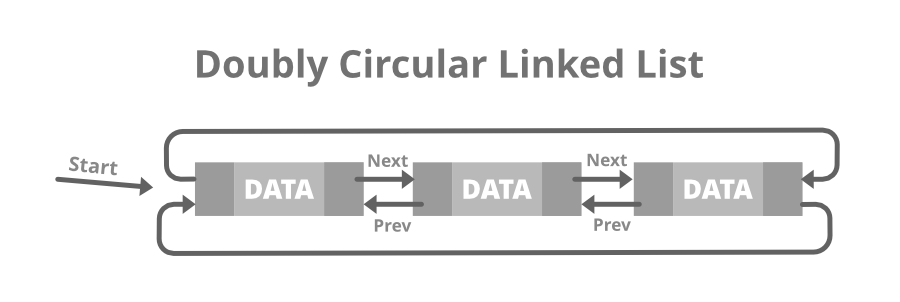Below is the structure of the Doubly Circular Linked List:

## C++

 `// Node of doubly circular linked list``struct` `Node {` `    ``int` `data;` `    ``// Pointer to next node in DCLL``    ``struct` `Node* next;` `    ``// Pointer to the previous node in DCLL``    ``struct` `Node* prev;``};`

## Java

 `// Structure of a Node``static` `class` `Node``{``    ``int` `data;``    ` `    ``// Pointer to next node in DCLL``    ``Node next;``    ` `    ``// Pointer to the previous node in DCLL``    ``Node prev;``};` `//this code is contributed by shivanisinghss2110`

## Python3

 `# structure of Node``class` `Node:``    ``def` `__init__(``self``, data):``        ``self``.previous ``=` `None``        ``self``.data ``=` `data``        ``self``.``next` `=` `None`

## C#

 `// Structure of a Node``public` `class` `Node``{``    ``public`  `int` `data;``    ` `    ``// Pointer to next node in DCLL``    ``public` `Node next;``    ` `    ``// Pointer to the previous node in DCLL``    ``public` `Node prev;``};` `// This code is contributed by shivanisinghss2110`

## Javascript

 ``

## C++

 `// C++ program to illustrate creation``// & traversal of Doubly Circular LL` `#include ``using` `namespace` `std;` `// Structure of a Node``struct` `Node {``    ``int` `data;``    ``struct` `Node* next;``    ``struct` `Node* prev;``};` `// Function to insert Node at``// the beginning of the List``void` `insertBegin(``struct` `Node** start, ``int` `value)``{``    ``// If the list is empty``    ``if` `(*start == NULL) {``        ``struct` `Node* new_node = ``new` `Node;``        ``new_node->data = value;``        ``new_node->next = new_node->prev = new_node;``        ``*start = new_node;``        ``return``;``    ``}` `    ``// Pointer points to last Node``    ``struct` `Node* last = (*start)->prev;` `    ``struct` `Node* new_node = ``new` `Node;` `    ``// Inserting the data``    ``new_node->data = value;` `    ``// Update the previous and``    ``// next of new node``    ``new_node->next = *start;``    ``new_node->prev = last;` `    ``// Update next and previous``    ``// pointers of start & last``    ``last->next = (*start)->prev = new_node;` `    ``// Update start pointer``    ``*start = new_node;``}` `// Function to traverse the circular``// doubly linked list``void` `display(``struct` `Node* start)``{``    ``struct` `Node* temp = start;` `    ``printf``(``"\nTraversal in"``           ``" forward direction \n"``);``    ``while` `(temp->next != start) {``        ``printf``(``"%d "``, temp->data);``        ``temp = temp->next;``    ``}``    ``printf``(``"%d "``, temp->data);` `    ``printf``(``"\nTraversal in "``           ``"reverse direction \n"``);``    ``Node* last = start->prev;``    ``temp = last;` `    ``while` `(temp->prev != last) {` `        ``// Print the data``        ``printf``(``"%d "``, temp->data);``        ``temp = temp->prev;``    ``}``    ``printf``(``"%d "``, temp->data);``}` `// Driver Code``int` `main()``{``    ``// Start with the empty list``    ``struct` `Node* start = NULL;` `    ``// Insert 5``    ``// So linked list becomes 5->NULL``    ``insertBegin(&start, 5);` `    ``// Insert 4 at the beginning``    ``// So linked list becomes 4->5``    ``insertBegin(&start, 4);` `    ``// Insert 7 at the end``    ``// So linked list becomes 7->4->5``    ``insertBegin(&start, 7);` `    ``printf``(``"Created circular doubly"``           ``" linked list is: "``);``    ``display(start);` `    ``return` `0;``}`

## Java

 `// Java program to illustrate creation``// & traversal of Doubly Circular LL` `import` `java.util.*;` `class` `GFG {` `    ``// Structure of a Node``    ``static` `class` `Node {``        ``int` `data;``        ``Node next;``        ``Node prev;``    ``};` `    ``// Start with the empty list``    ``static` `Node start = ``null``;` `    ``// Function to insert Node at``    ``// the beginning of the List``    ``static` `void` `insertBegin(``int` `value)``    ``{``        ``// If the list is empty``        ``if` `(start == ``null``) {``            ``Node new_node = ``new` `Node();``            ``new_node.data = value;``            ``new_node.next = new_node.prev = new_node;``            ``start = new_node;``            ``return``;``        ``}` `        ``// Pointer points to last Node``        ``Node last = (start).prev;` `        ``Node new_node = ``new` `Node();` `        ``// Inserting the data``        ``new_node.data = value;` `        ``// Update the previous and``        ``// next of new node``        ``new_node.next = start;``        ``new_node.prev = last;` `        ``// Update next and previous``        ``// pointers of start & last``        ``last.next = (start).prev = new_node;` `        ``// Update start pointer``        ``start = new_node;``    ``}` `    ``// Function to traverse the circular``    ``// doubly linked list``    ``static` `void` `display()``    ``{``        ``Node temp = start;` `        ``System.out.printf(``"\nTraversal in"``                          ``+ ``" forward direction \n"``);``        ``while` `(temp.next != start) {``            ``System.out.printf(``"%d "``, temp.data);``            ``temp = temp.next;``        ``}``        ``System.out.printf(``"%d "``, temp.data);` `        ``System.out.printf(``"\nTraversal in "``                          ``+ ``"reverse direction \n"``);``        ``Node last = start.prev;``        ``temp = last;` `        ``while` `(temp.prev != last) {` `            ``// Print the data``            ``System.out.printf(``"%d "``, temp.data);``            ``temp = temp.prev;``        ``}``        ``System.out.printf(``"%d "``, temp.data);``    ``}` `    ``// Driver Code``    ``public` `static` `void` `main(String[] args)``    ``{` `        ``// Insert 5``        ``// So linked list becomes 5.null``        ``insertBegin(``5``);` `        ``// Insert 4 at the beginning``        ``// So linked list becomes 4.5``        ``insertBegin(``4``);` `        ``// Insert 7 at the end``        ``// So linked list becomes 7.4.5``        ``insertBegin(``7``);` `        ``System.out.printf(``"Created circular doubly"``                          ``+ ``" linked list is: "``);``        ``display();``    ``}``}` `// This code is contributed by shikhasingrajput`

## Python3

 `# Python3 program to illustrate creation``# & traversal of Doubly Circular LL` `# structure of Node``class` `Node:``    ``def` `__init__(``self``, data):``        ``self``.previous ``=` `None``        ``self``.data ``=` `data``        ``self``.``next` `=` `None`  `class` `DoublyLinkedList:``    ``def` `__init__(``self``):``        ``self``.head ``=` `None``        ``self``.start_node ``=` `None``        ``self``.last_node ``=` `None` `    ``# function to add elements to doubly linked list``    ``def` `append(``self``, data):``        ``# is doubly linked list is empty then last_node will be none so in if condition head will be created``        ``if` `self``.last_node ``is` `None``:``            ``self``.head ``=` `Node(data)``            ``self``.last_node ``=` `self``.head``        ``# adding node to the tail of doubly linked list``        ``else``:``            ``new_node ``=` `Node(data)``            ``self``.last_node.``next` `=` `new_node``            ``new_node.previous ``=` `self``.last_node``            ``new_node.``next` `=` `self``.head``            ``self``.head.previous ``=` `new_node``            ``self``.last_node ``=` `new_node` `    ``# function to print the content of doubly linked list``    ``def` `display(``self``, ``Type``=``'Left_To_Right'``):``        ``if` `Type` `=``=` `'Left_To_Right'``:``            ``current ``=` `self``.head``            ``while` `current.``next` `is` `not` `None``:``                ``print``(current.data, end``=``' '``)``                ``current ``=` `current.``next``                ``if` `current ``=``=` `self``.head:``                    ``break``            ``print``()``        ``else``:``            ``current ``=` `self``.last_node``            ``while` `current.previous ``is` `not` `None``:``                ``print``(current.data, end``=``' '``)``                ``current ``=` `current.previous``                ``if` `current ``=``=` `self``.last_node.``next``:``                    ``print``(``self``.last_node.``next``.data, end``=``' '``)``                    ``break``            ``print``()`  `if` `__name__ ``=``=` `'__main__'``:``    ``L ``=` `DoublyLinkedList()``    ``L.append(``1``)``    ``L.append(``2``)``    ``L.append(``3``)``    ``L.append(``4``)``    ``L.display(``'Left_To_Right'``)``    ``L.display(``'Right_To_Left'``)`

## C#

 `// C# program to illustrate creation``// & traversal of Doubly Circular LL` `using` `System;` `public` `class` `GFG {` `    ``// Structure of a Node``    ``public` `        ``class` `Node {``        ``public` `            ``int` `data;``        ``public` `            ``Node next;``        ``public` `            ``Node prev;``    ``};` `    ``// Start with the empty list``    ``static` `Node start = ``null``;` `    ``// Function to insert Node at``    ``// the beginning of the List``    ``static` `void` `insertBegin(``int` `value)``    ``{``        ``Node new_node = ``new` `Node();` `        ``// If the list is empty``        ``if` `(start == ``null``) {` `            ``new_node.data = value;``            ``new_node.next = new_node.prev = new_node;``            ``start = new_node;``            ``return``;``        ``}` `        ``// Pointer points to last Node``        ``Node last = (start).prev;` `        ``// Inserting the data``        ``new_node.data = value;` `        ``// Update the previous and``        ``// next of new node``        ``new_node.next = start;``        ``new_node.prev = last;` `        ``// Update next and previous``        ``// pointers of start & last``        ``last.next = (start).prev = new_node;` `        ``// Update start pointer``        ``start = new_node;``    ``}` `    ``// Function to traverse the circular``    ``// doubly linked list``    ``static` `void` `display()``    ``{``        ``Node temp = start;` `        ``Console.Write(``"\nTraversal in"``                      ``+ ``" forward direction \n"``);``        ``while` `(temp.next != start) {``            ``Console.Write(temp.data + ``" "``);``            ``temp = temp.next;``        ``}``        ``Console.Write(temp.data + ``" "``);` `        ``Console.Write(``"\nTraversal in "``                      ``+ ``"reverse direction \n"``);``        ``Node last = start.prev;``        ``temp = last;` `        ``while` `(temp.prev != last) {` `            ``// Print the data``            ``Console.Write(temp.data + ``" "``);``            ``temp = temp.prev;``        ``}``        ``Console.Write(temp.data + ``" "``);``    ``}` `    ``// Driver Code``    ``public` `static` `void` `Main(String[] args)``    ``{` `        ``// Insert 5``        ``// So linked list becomes 5.null``        ``insertBegin(5);` `        ``// Insert 4 at the beginning``        ``// So linked list becomes 4.5``        ``insertBegin(4);` `        ``// Insert 7 at the end``        ``// So linked list becomes 7.4.5``        ``insertBegin(7);` `        ``Console.Write(``"Created circular doubly"``                      ``+ ``" linked list is: "``);``        ``display();``    ``}``}` `// This code is contributed by 29AjayKumar`

## Javascript

 ``

Output

```Created circular doubly linked list is:
Traversal in forward direction
7 4 5
Traversal in reverse direction
5 4 7```

So, in a header linked list START will not point to the first node of the list but START will contain the address of the header node. Below is the image for Grounded Header Linked List: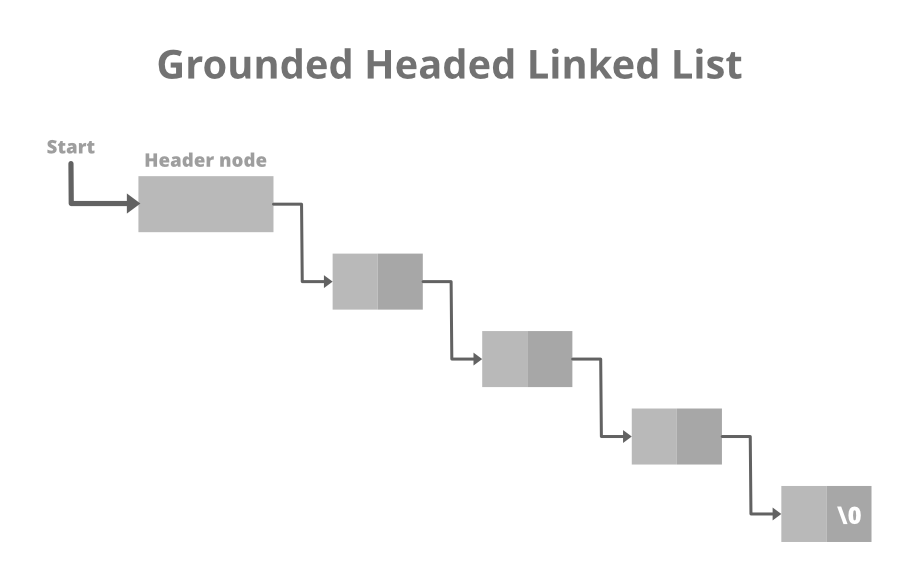## C++

 `// Structure of the list``struct` `link {``    ``int` `info;` `    ``// Pointer to the next node``    ``struct` `link* next;``};`

## Python3

 `# structure of Node``class` `Node:``    ``def` `__init__(``self``, data):``        ``self``.data ``=` `data``        ``self``.``next` `=` `None`

## Java

 `// Structure of the list``static` `class` `link {``    ``int` `info;``    ` `     ``// Pointer to the next node``    ``link next;``};` `// this code is contributed by shivanisinghss2110`

## C#

 `// Structure of the list``public` `class` `link {``    ``public` `int` `info;``    ` `    ``// Pointer to the next node``    ``public` `link next;``};` `// this code is contributed by shivanisinghss2110`

## Javascript

 ``

## C++

 `// C++ program to illustrate creation``// and traversal of Header Linked List` `#include ` `// Structure of the list``struct` `link {``    ``int` `info;``    ``struct` `link* next;``};` `// Empty List``struct` `link* start = NULL;` `// Function to create header of the``// header linked list``struct` `link* create_header_list(``int` `data)``{` `    ``// Create a new node``    ``struct` `link *new_node, *node;``    ``new_node = (``struct` `link*)``malloc``(``sizeof``(``struct` `link));``    ``new_node->info = data;``    ``new_node->next = NULL;` `    ``// If it is the first node``    ``if` `(start == NULL) {` `        ``// Initialize the start``        ``start = (``struct` `link*)``malloc``(``sizeof``(``struct` `link));``        ``start->next = new_node;``    ``}``    ``else` `{` `        ``// Insert the node in the end``        ``node = start;``        ``while` `(node->next != NULL) {``            ``node = node->next;``        ``}``        ``node->next = new_node;``    ``}``    ``return` `start;``}` `// Function to display the``// header linked list``struct` `link* display()``{``    ``struct` `link* node;``    ``node = start;``    ``node = node->next;` `    ``// Traverse until node is``    ``// not NULL``    ``while` `(node != NULL) {` `        ``// Print the data``        ``printf``(``"%d "``, node->info);``        ``node = node->next;``    ``}``    ``printf``(``"\n"``);` `    ``// Return the start pointer``    ``return` `start;``}` `// Driver Code``int` `main()``{``    ``// Create the list``    ``create_header_list(11);``    ``create_header_list(12);``    ``create_header_list(13);` `    ``// Print the list``    ``printf``(``"List After inserting"``           ``" 3 elements:\n"``);``    ``display();``    ``create_header_list(14);``    ``create_header_list(15);` `    ``// Print the list``    ``printf``(``"List After inserting"``           ``" 2 more elements:\n"``);``    ``display();` `    ``return` `0;``}`

## Java

 `// Java program to illustrate creation``// and traversal of Header Linked List` `class` `GFG {``    ``// Structure of the list``    ``static` `class` `link {``        ``int` `info;``        ``link next;``    ``};` `    ``// Empty List``    ``static` `link start = ``null``;` `    ``// Function to create header of the``    ``// header linked list``    ``static` `link create_header_list(``int` `data)``    ``{` `        ``// Create a new node``        ``link new_node, node;``        ``new_node = ``new` `link();``        ``new_node.info = data;``        ``new_node.next = ``null``;` `        ``// If it is the first node``        ``if` `(start == ``null``) {` `            ``// Initialize the start``            ``start = ``new` `link();``            ``start.next = new_node;``        ``}``        ``else` `{` `            ``// Insert the node in the end``            ``node = start;``            ``while` `(node.next != ``null``) {``                ``node = node.next;``            ``}``            ``node.next = new_node;``        ``}``        ``return` `start;``    ``}` `    ``// Function to display the``    ``// header linked list``    ``static` `link display()``    ``{``        ``link node;``        ``node = start;``        ``node = node.next;` `        ``// Traverse until node is``        ``// not null``        ``while` `(node != ``null``) {` `            ``// Print the data``            ``System.out.printf(``"%d "``, node.info);``            ``node = node.next;``        ``}``        ``System.out.printf(``"\n"``);` `        ``// Return the start pointer``        ``return` `start;``    ``}` `    ``// Driver Code``    ``public` `static` `void` `main(String[] args)``    ``{``        ``// Create the list``        ``create_header_list(``11``);``        ``create_header_list(``12``);``        ``create_header_list(``13``);` `        ``// Print the list``        ``System.out.printf(``"List After inserting"``                          ``+ ``" 3 elements:\n"``);``        ``display();``        ``create_header_list(``14``);``        ``create_header_list(``15``);` `        ``// Print the list``        ``System.out.printf(``"List After inserting"``                          ``+ ``" 2 more elements:\n"``);``        ``display();``    ``}``}` `// This code is contributed by 29AjayKumar`

## Python3

 `# Python3 program to illustrate creation``# and traversal of Header Linked List` `# structure of Node``class` `Node:``    ``def` `__init__(``self``, data):``        ``self``.data ``=` `data``        ``self``.``next` `=` `None`  `class` `LinkedList:``    ``def` `__init__(``self``):``        ``self``.head ``=` `Node(``0``)``        ``self``.last_node ``=` `self``.head` `    ``# function to add elements to header linked list``    ``def` `append(``self``, data):``        ``self``.last_node.``next` `=` `Node(data)``        ``self``.last_node ``=` `self``.last_node.``next` `    ``# function to print the content of header linked list``    ``def` `display(``self``):``        ``current ``=` `self``.head.``next``        ``# traversing the header linked list``        ``while` `current ``is` `not` `None``:``            ``# at each node printing its data``            ``print``(current.data, end``=``' '``)``            ``# giving current next node``            ``current ``=` `current.``next``        ``# print(self.head.data)``        ``print``()` `# Driver code``if` `__name__ ``=``=` `'__main__'``:``    ``L ``=` `LinkedList()``    ``# adding elements to the header linked list``    ``L.append(``1``)``    ``L.append(``2``)``    ``L.append(``3``)``    ``L.append(``4``)``    ``# displaying elements of header linked list``    ``L.display()`

## C#

 `// C# program to illustrate creation``// and traversal of Header Linked List` `using` `System;` `public` `class` `GFG {``    ``// Structure of the list``    ``public` `class` `link {``        ``public` `int` `info;``        ``public` `link next;``    ``};` `    ``// Empty List``    ``static` `link start = ``null``;` `    ``// Function to create header of the``    ``// header linked list``    ``static` `link create_header_list(``int` `data)``    ``{` `        ``// Create a new node``        ``link new_node, node;``        ``new_node = ``new` `link();``        ``new_node.info = data;``        ``new_node.next = ``null``;` `        ``// If it is the first node``        ``if` `(start == ``null``) {` `            ``// Initialize the start``            ``start = ``new` `link();``            ``start.next = new_node;``        ``}``        ``else` `{` `            ``// Insert the node in the end``            ``node = start;``            ``while` `(node.next != ``null``) {``                ``node = node.next;``            ``}``            ``node.next = new_node;``        ``}``        ``return` `start;``    ``}` `    ``// Function to display the``    ``// header linked list``    ``static` `link display()``    ``{``        ``link node;``        ``node = start;``        ``node = node.next;` `        ``// Traverse until node is``        ``// not null``        ``while` `(node != ``null``) {` `            ``// Print the data``            ``Console.Write(``"{0} "``, node.info);``            ``node = node.next;``        ``}``        ``Console.Write(``"\n"``);` `        ``// Return the start pointer``        ``return` `start;``    ``}` `    ``// Driver Code``    ``public` `static` `void` `Main(String[] args)``    ``{``        ``// Create the list``        ``create_header_list(11);``        ``create_header_list(12);``        ``create_header_list(13);` `        ``// Print the list``        ``Console.Write(``"List After inserting"``                      ``+ ``" 3 elements:\n"``);``        ``display();``        ``create_header_list(14);``        ``create_header_list(15);` `        ``// Print the list``        ``Console.Write(``"List After inserting"``                      ``+ ``" 2 more elements:\n"``);``        ``display();``    ``}``}` `// This code is contributed by 29AjayKumar`

## Javascript

 ``

Output

```List After inserting 3 elements:
11 12 13
List After inserting 2 more elements:
11 12 13 14 15```